# Categories for Quantum#

Alexis Toumi & Giovanni de Felice

5th May 2021, Tallcat

1. Categorical quantum mechanics

• the miracle of scalars

• the way of the dagger

• braids and symmetry

• compact closed categories

• special commutative Frobenius algebras

• bialgebras & Hopf algebras

2. Qubit quantum computing

• the circuit model

• the ZX-calculus

3. Applications

• optimisation

• compilation

• extraction

• natural language processing

## Categorical quantum mechanics#

The traditional formulation of quantum theory happens in $$\mathbf{Hilb}$$, the monoidal category of Hilbert spaces and bounded linear maps with the tensor product.

Categorical quantum mechanics (CQM) seeks to make explicit precisely what structure is needed of $$\mathbf{Hilb}$$ in order to recover quantum theory.

References:

• A categorical semantics of quantum protocols, Abramsky & Coecke (arXiv:0402130)

• Categorical quantum mechanics, Abramsky & Coecke (arXiv:0808.1023)

• Picturing quantum processes: a first course in quantum theory and diagrammatic reasoning, Coecke & Kissinger (2017)

• Categories for quantum theory: an introduction, Heunen & Vicary (2019)

In the process, we get a diagrammatic language for quantum processes.

We also get a new intuition for monoidal categories:

• Systems are objects $$A, B$$, composition of systems is given by their tensor product $$A \otimes B$$.

• Processes are arrows $$f : A \to B$$, $$g : B \to C$$, with their sequential and parallel compositions $$g \circ f$$ and $$f \otimes g$$.

• States are arrows from the unit $$v : 1 \to A$$.

• Effects are arrows into the unit $$e : A \to 1$$.

• Scalars are endomorphisms of the unit $$s : 1 \to 1$$.

### The miracle of scalars#

Lemma: Tensoring with a scalar is a natural transformation.

Proof:

:

from discopy.monoidal import Ty, Box
from discopy.drawing import Equation

x, y, z = Ty(*"xyz")
f, g = Box('f', x, y), Box('g', y, z)
a, b, c, d = (Box(x, Ty(), Ty()) for x in 'abcd')

assert a @ x >> f == (f >> a @ y).normal_form()
Equation(a @ x >> f, f >> a @ y).draw(figsize=(5, 2))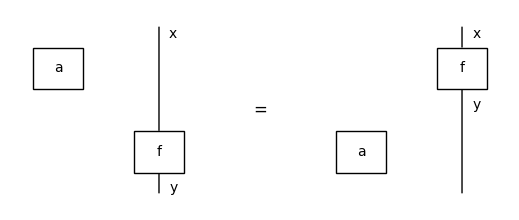Lemma: In a monoidal category, scalars form a commutative monoid.

Proof: Eckmann-Hilton argument.

:

from discopy import drawing

assert (a @ b).interchange(0, 1) == b @ a
assert (a @ b).interchange(0, 1).interchange(0, 1) == a @ b

drawing.to_gif(a @ b, b @ a, loop=True, figsize=(.5, 1), path='../_static/monoidal/Eckmann-Hilton.gif')

: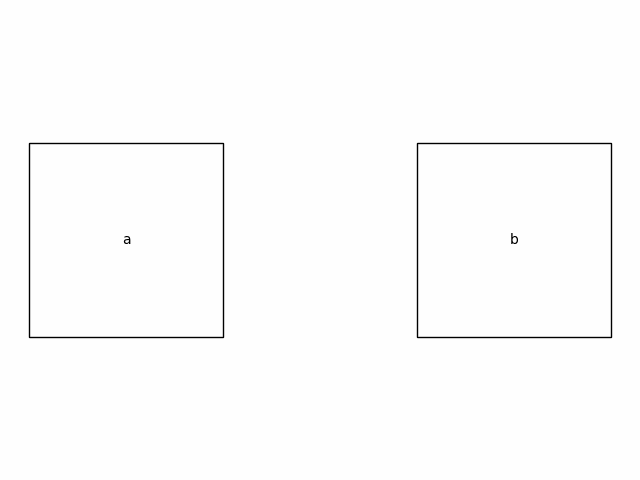:Definition: A monoidal category is enriched in commutative monoids (com.mon. enriched) if every homset is a commutative monoid such that composition and tensor are monoid homomorphisms.

$(f + f') \otimes (g + g') = (f \otimes g) + (f' \otimes g) + (f \otimes g') + (f' \otimes g')$
$(f + f') \circ (g + g') = (f \circ g) + (f' \circ g) + (f \circ g') + (f' \circ g')$
$0 \otimes f = 0 \circ f = 0 = f \circ 0 = f \otimes 0$
:

from discopy.monoidal import Sum

zero = Sum((), Ty(), Ty())

assert zero + a == Sum((a, )) == a + zero
assert (a + b) + c == a + b + c == a + (b + c)
assert (a + b) @ (c + d) == (a @ c) + (a @ d) + (b @ c) + (b @ d)
assert (a + b) >> (c + d) == (a >> c) + (a >> d) + (b >> c) + (b >> d)


Lemma: In a com.mon. enriched monoidal category, the scalars form a commutative semiring.

Definition: A commutative semiring is a com.mon. enriched monoidal category with one object.

Lemma: Given a commutative semiring $$\mathbb{S}$$, the category $$\mathbf{Mat}_\mathbb{S}$$ of $$\mathbb{S}$$-valued matrices is monoidal with the Kronecker product.

Lemma: $$\mathbf{FHilb} \simeq \mathbf{Mat}_\mathbb{C}$$.

Lemma: $$\mathbf{FRel} \simeq \mathbf{Mat}_\mathbb{B}$$.

Com.mon. enrichment allows us to talk about superposition, basis and dimension in an abstract setting.

Definition: Given two states $$v, w : 1 \to A$$, their superposition is a linear combination $$a \otimes v + b \otimes w : 1 \to A$$ for two scalars $$a, b : 1 \to 1$$.

Definition: A basis for $$A$$ is a minimal set of states $$\{\vert i \rangle : 1 \to A\}$$ such that every state $$w : 1 \to A$$ is a linear combination $$w = \sum_i a_i \otimes \vert i \rangle$$ for some scalars $$a_i : 1 \to 1$$.

Definition: The dimension of a system is the cardinality of its smallest basis.

### The way of the dagger#

Definition: A dagger is a contravariant involutive identity-on-objects monoidal functor.

In terms of diagrams, the dagger is the vertical reflexion.

We may draw asymmetric boxes to distinguish a generator from its dagger.

:

Equation(f, f[::-1], symbol="$\mapsto$").draw(asymmetry=.25, figsize=(3, 1))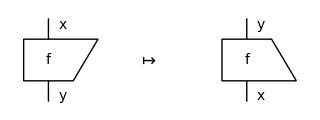:

from discopy.monoidal import Id

assert Id(x)[::-1] == Id(x)
assert (f >> g)[::-1] ==  g[::-1] >> f[::-1]
assert (f @ g)[::-1].normal_form() ==  f[::-1] @ g[::-1]

Equation((f @ g)[::-1], f[::-1] @ g[::-1]).draw(figsize=(5, 2))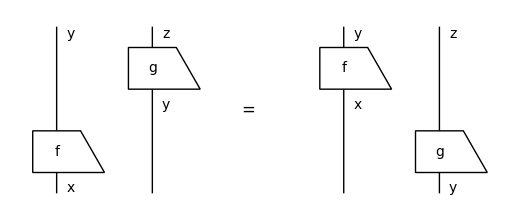The dagger allows us to formulate the notion of inner product in an abstract setting.

Definition: The inner product of two states $$w, w' : 1 \to A$$ is the scalar $$w^\dagger \circ w' : 1 \to 1$$.

Definition: The squared norm of a state $$w : 1 \to A$$ is the scalar $$w^\dagger \circ w$$. It is normalised when it has norm $$1$$, the identity of the monoidal unit.

Definition: Two states $$w, w' : 1 \to A$$ are orthogonal when $$w^\dagger \circ w' = 0$$.

Definition: An isometry is a norm-preserving process $$f : A \to B$$, i.e. $$f^\dagger \circ f = \text{id}_A$$.

Definition: A unitary is an isomorphism $$f : A \to B$$ with inverse $$f^\dagger$$, i.e. $$f^\dagger \circ f = \text{id}_A$$ and $$f \circ f^\dagger = \text{id}_B$$.

Definition: A basis is orthonormal if $$\langle i | j \rangle = \delta_{ij}$$.

It also allows us to talk about quantum measurements, the Born rule and even a simple form of Heisenberg’s uncertainty principle!

Definition: A projector is a process $$p : A \to A$$ such that $$p = p^\dagger$$ and $$p \circ p = \text{id}_A$$.

Example: Take $$w \circ w^\dagger : A \to A$$ for any normalised state $$w : 1 \to A$$.

Definition: A (projection-valued) measurement is a set of projectors $$\{p_i : A \to A\}$$ such that $$\sum p_i = \text{id}_A$$.

Example: Take an orthonormal basis $$\{\vert i \rangle\}$$ then $$\{\vert i \rangle \langle i \vert\}$$ is a measurement.

Lemma: A measurement is a choice of basis and a partition of it.

Definition (Born rule): Given a normalised state $$w : 1 \to A$$ and a measurement $$\{p_i : A \to A\}$$, the probability of measuring outcome $$i$$ and getting the state $$p_i(w)$$ as a result is $$P(i \vert w) = w^\dagger \circ p_i \circ w$$.

Example: Take $$p_i = v \circ v^\dagger$$ then we get the squared amplitude $$P(v \vert w) = \langle w \vert v \rangle^2$$.

Definition: Two bases are complementary (a.k.a. mutually unbiased) if $$P(i \vert j) = P(i' \vert j')$$ for all $$i, i'$$ and $$j, j'$$.

Example: Take $$\{\vert 0 \rangle, \vert 1 \rangle\}$$ and $$\{\vert + \rangle, \vert - \rangle\}$$ the two bases of $$\mathbb{C}^2$$ with:

$\vert + \rangle = \frac{\vert 0 \rangle + \vert 1 \rangle}{\sqrt{2}} \quad and \quad \vert - \rangle = \frac{\vert 0 \rangle - \vert 1 \rangle}{\sqrt{2}}$

.

### Braids and symmetry#

Definition: A (strict) monoidal category is braided if for every objects $$A, B$$ we have swaps: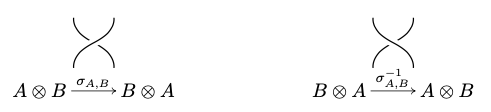such that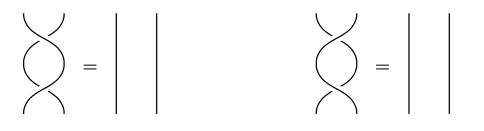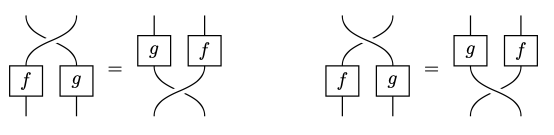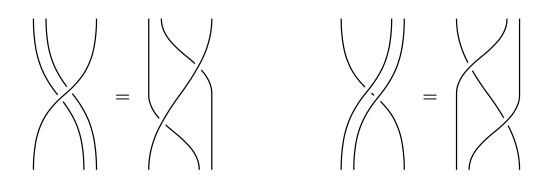Definition: A braided monoidal category is symmetric if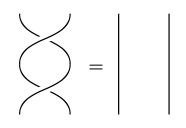or equivalently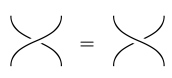Lemma: In a braided monoidal category, we can prove the Yang-Baxter equation.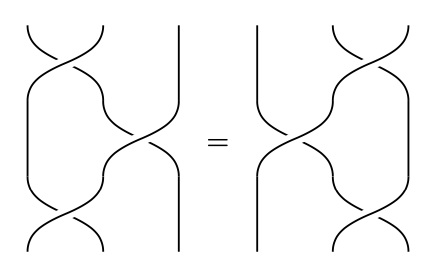Proof: Naturality + Hexagon.

### Compact closed categories#

Recall: In a right-rigid monoidal category, for every $$L$$ there is a right adjoint $$R$$ and a pair of arrows: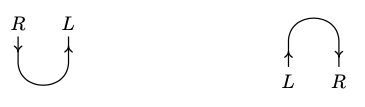such that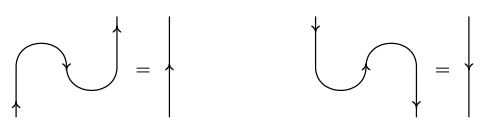Lemma: In a braided monoidal category, left and right adjoints collapse.

Proof: Define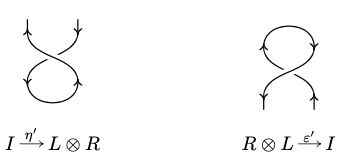Then from naturality we get: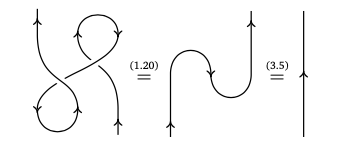Definition: A compact closed category is a rigid symmetric monoidal category.

Lemma: In a compact closed category, the following equations hold: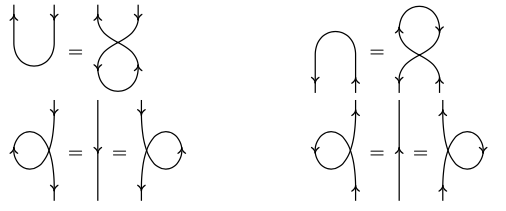Definition: The transpose of a process is given by: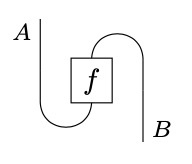Definition: A $$\dagger$$ compact closed category is a compact closed category with a dagger, such that swaps are unitaries and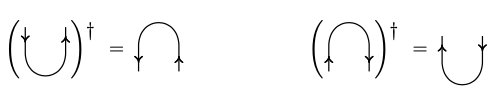In a $$\dagger$$ compact closed category, we get a $$\mathbb{Z}_2 \times \mathbb{Z}_2$$ action on processes: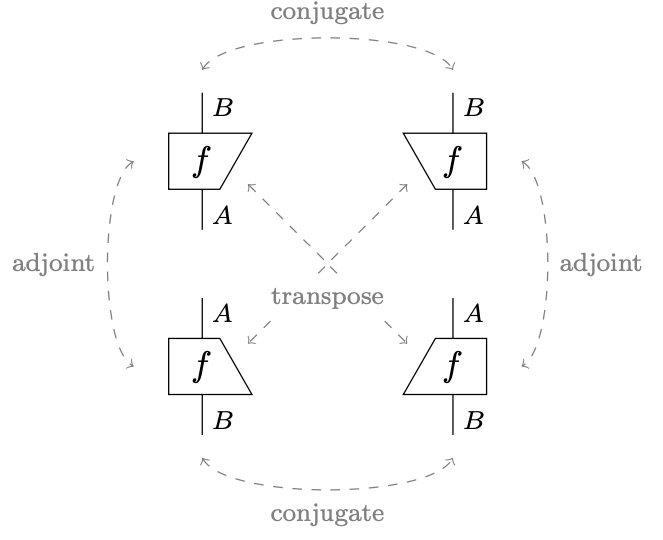Compact closed categories allow to formulate the notion of entanglement in an abstract setting.

Definition: A state $$w : 1 \to A \otimes B$$ is separable when it can be written as a tensor $$w = v \otimes v'$$ for some $$v : 1 \to A$$ and $$v' 1 \to B$$.

Definition: A state $$w : 1 \to A \otimes B$$ is entangled if it is not separable.

Lemma: The cup of a non-trivial system is entangled.

Proof: Suppose the cup is separable, then the identity factors through the unit.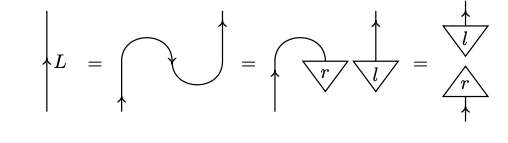Lemma (Teleportation):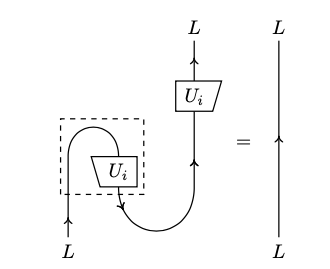Proof: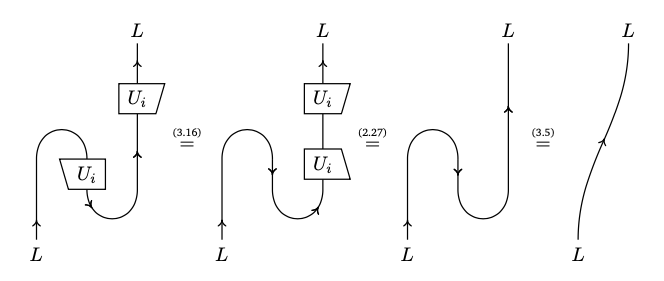We can also formulate an abstract version of the no-deleting and no-cloning theorem.

Definition: Deleting is a natural transformation $$e_A : A \to 1$$ with $$e_1 = 1$$.

Lemma: A monoidal category has deleting if and only if $$1$$ is terminal.

Theorem: If a compact closed category has deleting, then it is a preorder.

Proof: Take two parallel arrows $$f, g : A \to B$$, then their coname $$\hat f, \hat g : A \otimes B^\star \to 1$$ are equal, hence $$f = g$$.

Definition: Copying is an commutative, associative natural transformation $$d_A : A \to A \otimes A$$ such that: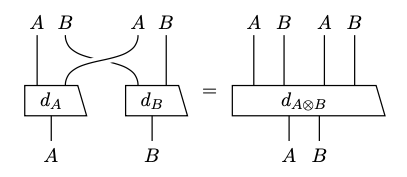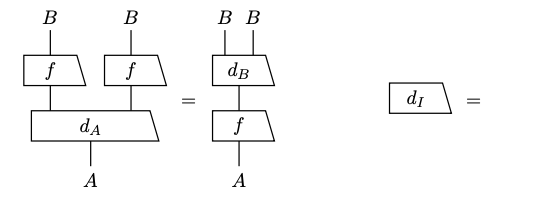Lemma: If a braided monoidal category has copying, then: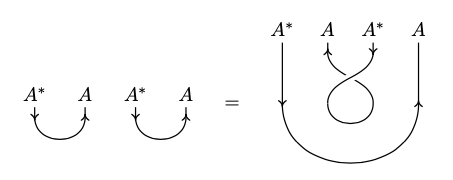Proof: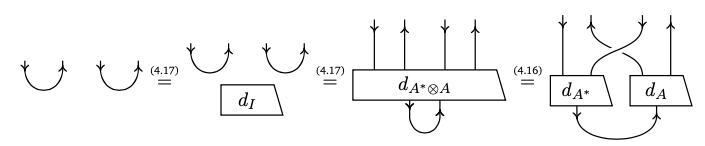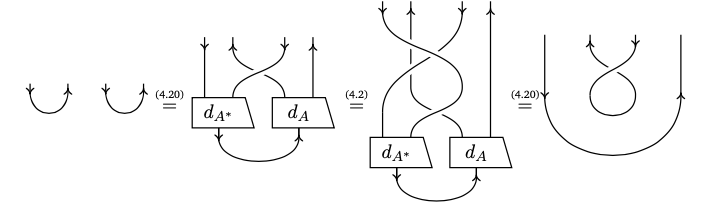Theorem: If a compact closed category has copying, then: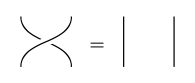Proof: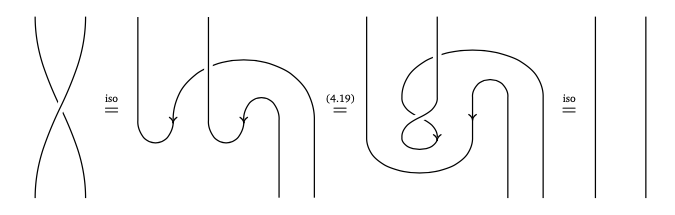### Special commutative Frobenius algebras#

Definition: A Frobenius algebra is a pair of a monoid and comonoid such that: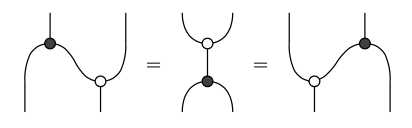Definition: A Frobenius algebra is dagger when the dagger of multiplication is comultiplication, and the dagger of unit is counit.

Definition: A $$\dagger$$ Frobenius algebra is commutative when its monoid is commutative.

Definition: A $$\dagger$$ Frobenius algebra is special when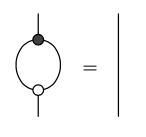Proof: Define cups and caps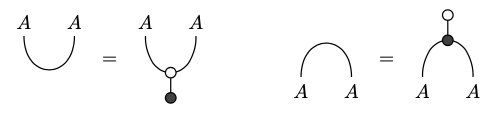then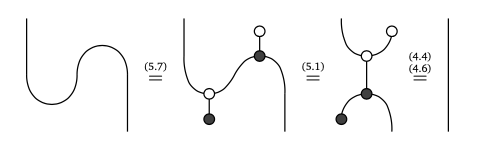Lemma: Homomorphisms of Frobenius algebras are isomorphisms, with inverse given by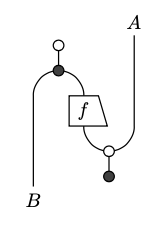Proof: Suppose $$f$$ is a homomorphism of Frobenius algebras.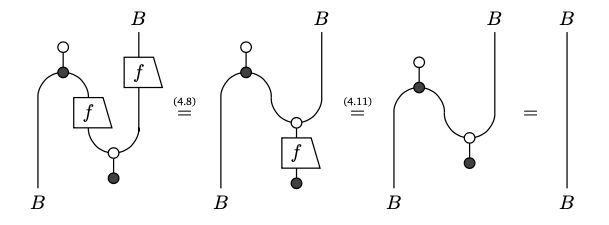Theorem (Spider Fusion): Every connected diagram $$d : A^{\otimes n} \to A^{\otimes m}$$ generated by the co/monoid of a Frobenius algebra is equal to a spider-shaped diagram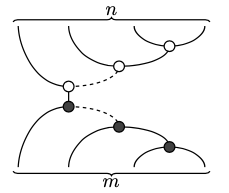Proof: By induction, we move all the black comonoid below the white monoid.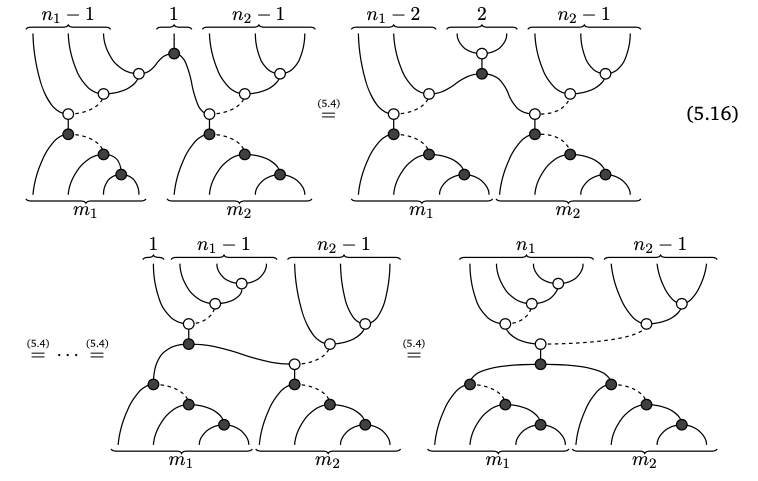Theorem (Artin–Wedderburn): in $$\mathbf{FHilb}$$, $$\dagger$$ special commutative Frobenius algebras are in one-to-one correspondance with orthonormal bases.

Proof: $$C^\star$$ algebra magic.

The correspondance sends a $$\dagger$$ SCFA to the basis of copyable states: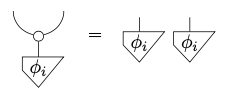It sends a basis to the Frobenius algebra given by: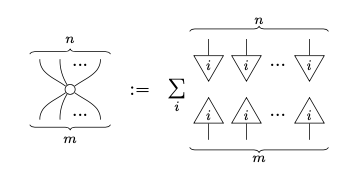Frobenius algebras not only characterise bases in $$\mathbf{Hilb}$$, they also allow us to formulate an abstract notion of phases.

Definition: Given a $$\dagger$$SCFA, a phase is a state $$a : 1 \to A$$ such that: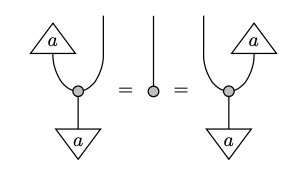It defines a phase shift map, a.k.a. diagonal unitary matrices: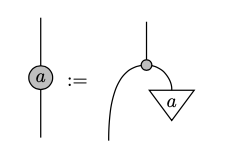Lemma: Phases form a group.